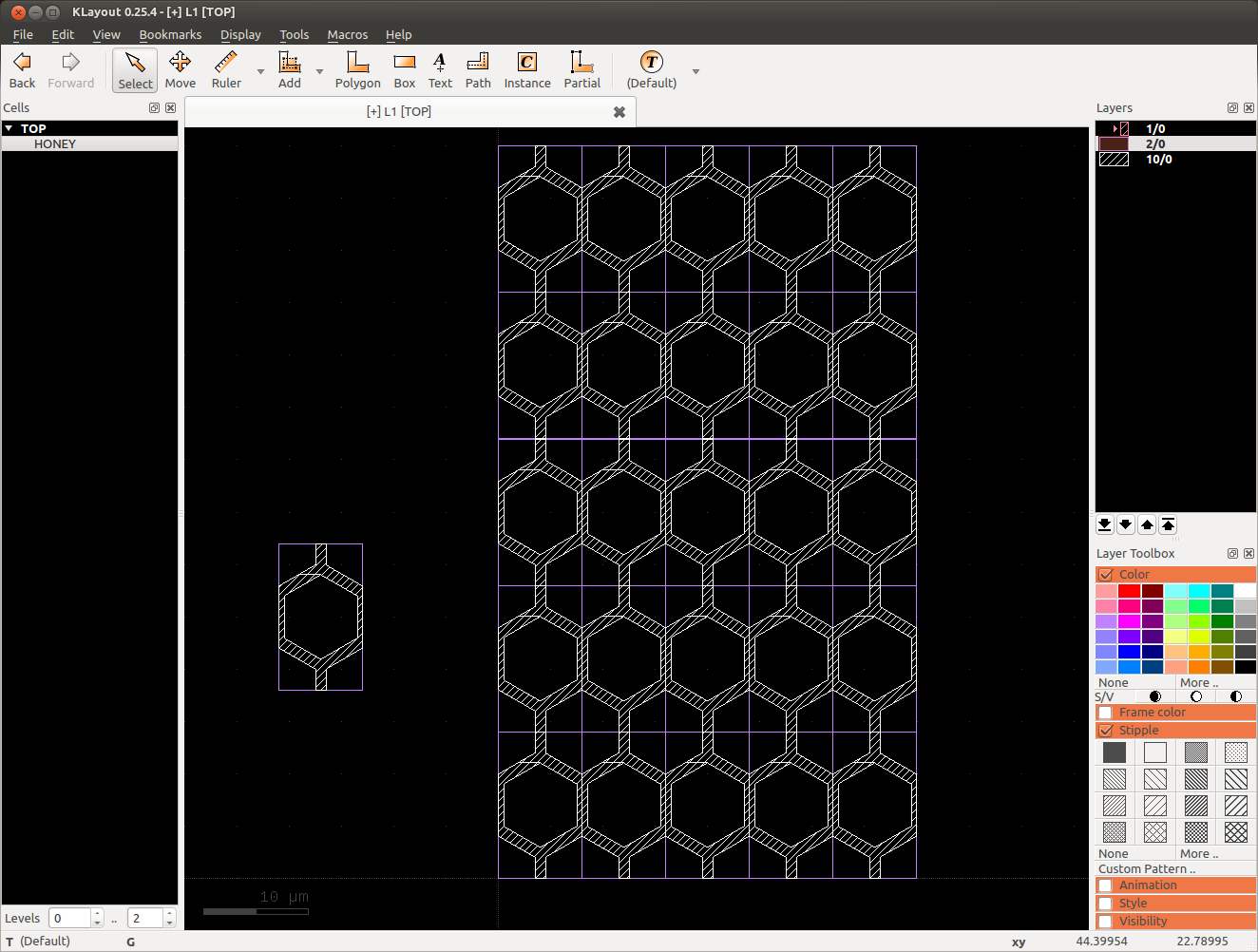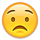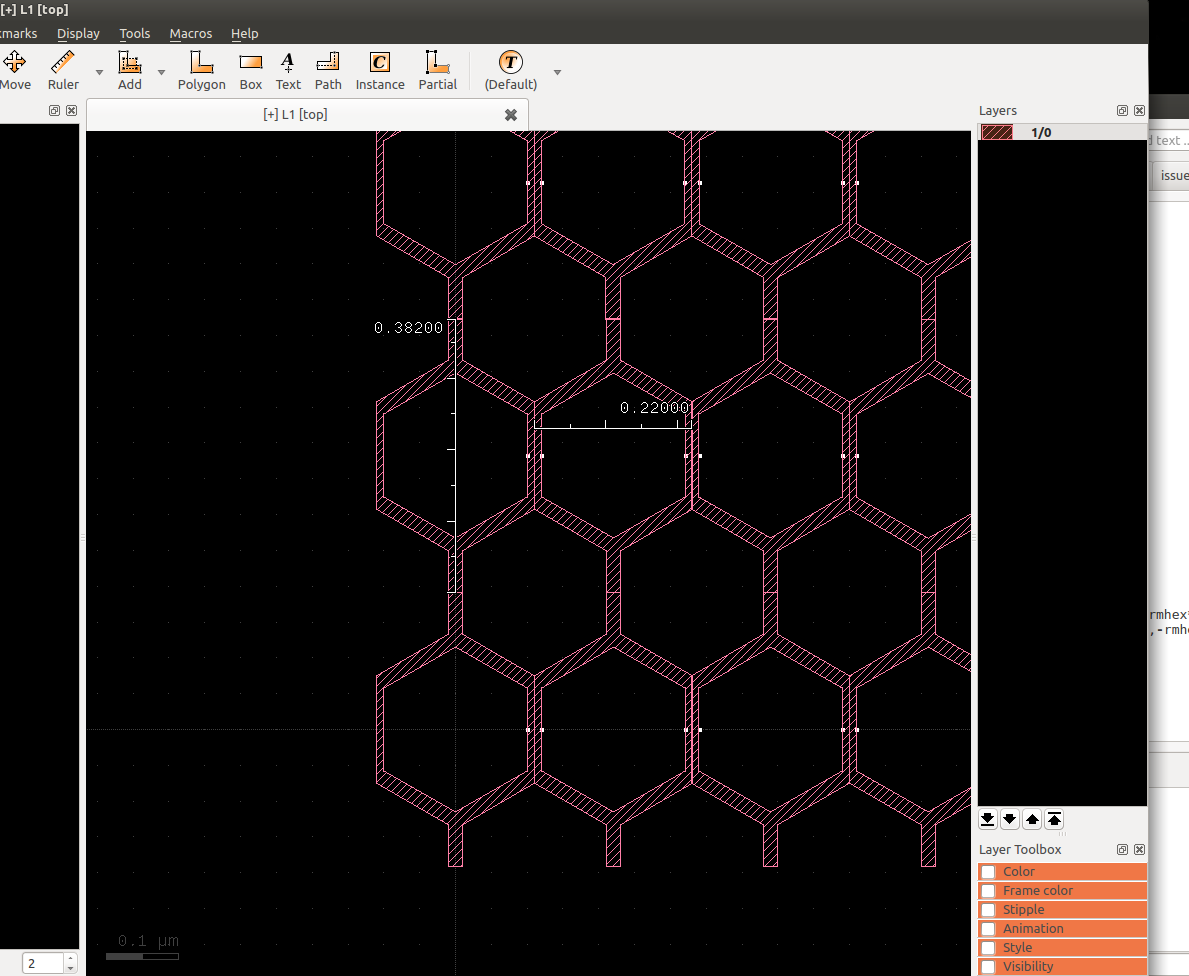#### Howdy, Stranger!

It looks like you're new here. If you want to get involved, click one of these buttons!

# HELP: HoneyComb with Circle Pcell

edited January 11

Hi,

I'm trying to learn to create a Honeycomb PCell based on the circle example we have in Ruby (I didn't find the same example in Python).

The Circle function in Klayout in Basic works differently than the one in the example.

If I choose Instance -> Basic Lib -> Circle, than to create an Hexagon I change the Number of points to "6".
The distance between the 2 edge of the Hexagon equals to the 2*Radius defined in the PCell.

In the case of the circle example in Ruby, the distance will be by default the 2 * Radius * sqrt(3/4) of the Hexagon.
My target is to write a Pcell where I can define the Width of the Honeycomb and the Thickness of the wall, then adding the number repetition in line and in column as parameters.

I'm thinking to use the difference between two Hexagon (circle of 6 points) of difference radius.

Any suggestion to how to write the code in Ruby or Python will be very appreciated.

Thank you.

• edited January 10

After verifying the result, I think The basic Circle create a polygon tan to the outside of the circle for 6 points. The ruby code of the circle, create the polygon on the inside of the circle.

Any help with the code will be welcome.
Thank you.

• I was able to create a PCell to do one cell of the Honeycomb. I don't know how I can create an array in rectangular shape without flattening the PCell.

Any idea?

• Any help?
• Hi,

I think the problem is that the array feature will not allow you to create a rectangular area filled with honeycombs. Will it help, if the basic cell is a rectangular cross section of the honeycomb as in this screenshot?

Matthias• Great idea. I will try to create a Pcell of this shape and use the array feature to create the final shape.

Sometimes the solution is more easy than what I was expectedBig thank Matthias. Hopefully, I will be able to create a Pcell with the suggestion shape.

• edited January 16

I failed to write the script in PythonBut I was able to reproduce the same result if I create the cell manually (less precise) directly in the display windows environment.

If you wish, I can send you the code I wrote and show me how to fix it.

• Yes, please do. Ideally you'd attach it here or put it on a gist and link it here. This is a place where everyone can learn and this is a opportunity to do so.

Matthias

• # The code

I'm not sure yet how to create the outside polygon correctly.
I tried also to create a circle where I multiply the radius by sqrt(3/4) to have the internal distance exactly equal to the defined radius.

The final target is to create honeycombs cell to fit in a rectangular shape.
The 4 parameters I need to defined are:

• The internal gap
• The wall thickness
• The Width of the rectangle
• The High of the rectangle

``````import pya
import math

class HoneyCombs(pya.PCellDeclarationHelper):

def __init__(self):
# Important: initialize the super class
super(HoneyCombs, self).__init__()
# declare the parameters
self.param("l", self.TypeLayer, "Layer", default = pya.LayerInfo(1, 0))
self.param("hi", self.TypeShape, "", default = pya.DPoint(5, 0))
self.param("ho", self.TypeShape, "", default = pya.DPoint(10, 0))
self.param("ri", self.TypeDouble, "Inner radius", default = 0.1)
self.param("ro", self.TypeDouble, "Outer radius", default = 0.12)
self.param("wt", self.TypeDouble, "Wall Thickness", default = 20, readonly = True)
self.param("ri_", self.TypeDouble, "Inner radius", default = 5, hidden = True)
self.param("ro_", self.TypeDouble, "Outer radius", default = 10, hidden = True)
self.param("h", self.TypeDouble, "Head length", default = 4, hidden = True)
self.param("w", self.TypeDouble, "Body width", default = 1, hidden = True)

def display_text_impl(self):
return "HoneyCombs(Ri=" + str('%.1f' % self.ri) + ", Ro=" + ('%.1f' % self.ro) +")"

def coerce_parameters_impl(self):
self.wt = self.ro-self.ri
self.h=self.ro-self.ri
self.w=self.ri*4*math.sqrt(3/4)
if self.ri < 0:
self.ri *= -1
if self.ro < 0:
self.ro *= -1
if self.ri_ != self.ri or self.ro_ != self.ro:
# update handle
self.hi = pya.DPoint(self.ri, 0)
self.ho = pya.DPoint(self.ro, 0)
# fix params
self.ri_ = self.ri
self.ro_ = self.ro
else:
# calc params from handle
self.ri = self.ri_ = math.sqrt(abs(self.hi.x)**2+abs(self.hi.y)**2)
self.ro = self.ro_ = math.sqrt(abs(self.ho.x)**2+abs(self.ho.y)**2)

def can_create_from_shape_impl(self):
return self.shape.is_box() or self.shape.is_polygon() or self.shape.is_path()

def parameters_from_shape_impl(self):
self.ro = self.shape.bbox().width() * self.layout.dbu / 2
self.ri = self.ro/2
self.l = self.layout.get_info(self.layer)

def transformation_from_shape_impl(self):
return pya.Trans(self.shape.bbox().center())

def produce_impl(self):
dbu = self.layout.dbu
self.ri = self.ri / math.sqrt(3/4)
self.ro = self.ro / math.sqrt(3/4)
hexi = []
for i in range(6):
angle = i*anglestep
x = self.ri*math.cos(angle)
y = self.ri*math.sin(angle)
hexi.append(pya.Point.from_dpoint(pya.DPoint(x/dbu, y/dbu)))
hexo = []
for i in range(6):
angle = i*anglestep
x = self.ro*math.cos(angle)
y = self.ro*math.sin(angle)
hexo.append(pya.Point.from_dpoint(pya.DPoint(x/dbu, y/dbu)))
comb1 = []
comb1.append(pya.Point.from_dpoint(pya.DPoint(self.w/2/dbu, -self.h/2/dbu)))
comb1.append(pya.Point.from_dpoint(pya.DPoint(self.w/2/dbu, self.h/2/dbu)))
comb1.append(pya.Point.from_dpoint(pya.DPoint(self.ri/dbu, self.h/2/dbu)))
comb1.append(pya.Point.from_dpoint(pya.DPoint(self.ri/dbu, -self.h/2/dbu)))
comb2 = []
comb2.append(pya.Point.from_dpoint(pya.DPoint(-self.ri/dbu, -self.h/2/dbu)))
comb2.append(pya.Point.from_dpoint(pya.DPoint(-self.ri/dbu, self.h/2/dbu)))
comb2.append(pya.Point.from_dpoint(pya.DPoint(-self.w/2/dbu, self.h/2/dbu)))
comb2.append(pya.Point.from_dpoint(pya.DPoint(-self.w/2/dbu, -self.h/2/dbu)))

#      x = self.ro*math.cos(angle)
#      y = self.ro*math.sin(angle)
#      star.append(pya.Point.from_dpoint(pya.DPoint(x/dbu, y/dbu)))
comb1 = pya.Polygon(comb1)
comb2 = pya.Polygon(comb2)
hexo = pya.Polygon(staro)
hexo.insert_hole(hexi)
self.cell.shapes(self.l_layer).insert(pya.Polygon(hexo))
self.cell.shapes(self.l_layer).insert(pya.Polygon(comb1))
self.cell.shapes(self.l_layer).insert(pya.Polygon(comb2))

class MyLib(pya.Library):

def __init__(self):

# Set the description
self.description = "My First Library"

# Create the PCell declarations
self.layout().register_pcell("Poly_donut", Poly_donut())
self.layout().register_pcell("HoneyCombs", HoneyCombs())
# That would be the place to put in more PCells ...

# Register us with the name "MyLib".
# If a library with that name already existed, it will be replaced then.
self.register("MyLib")

# Instantiate and register the library
MyLib()
``````
• edited January 21

sorry for the late reply - your code isn't bad. Thanks for sharing it. There is a little redundancy with the hidden parameters, but unless you strive for maximum compatibility, that's fine.

I have changed the generator code to match the screenshot above:

``````  def produce_impl(self):
dbu = self.layout.dbu
mu2dbu = pya.DCplxTrans(dbu).inverted()
rihex = self.ri / math.sqrt(3/4)
rohex = self.ro / math.sqrt(3/4)
rmhex = 0.5*(rihex+rohex)
hexi = []
for i in range(6):
angle = i*anglestep
x = rihex*math.sin(angle)
y = rihex*math.cos(angle)
hexi.append(mu2dbu*pya.DPoint(x, y))
hexo = []
for i in range(6):
angle = i*anglestep
x = rohex*math.sin(angle)
y = rohex*math.cos(angle)
hexo.append(mu2dbu*pya.DPoint(x, y))
rm = 0.5*(self.ri+self.ro)
clipbox = pya.Box(mu2dbu*pya.DPoint(-rm,-2*rm),mu2dbu*pya.DPoint(rm,2*rm))
bartop = pya.Box(mu2dbu*pya.DPoint(-0.5*self.wt,rihex),mu2dbu*pya.DPoint(0.5*self.wt,rmhex*3/2))
barbot = pya.Box(mu2dbu*pya.DPoint(-0.5*self.wt,-rihex),mu2dbu*pya.DPoint(0.5*self.wt,-rmhex*3/2))

# Use a region to produce the result
res = pya.Region(pya.Polygon(hexo))
res -= pya.Region(pya.Polygon(hexi))
res += bartop
res += barbot
res &= clipbox
res.merge()
self.cell.shapes(self.l_layer).insert(res)
``````

Please note that I use Region objects to compute the final polygon. Regions offer boolean operations which come handy here.

You see below how the result looks like. I manually created the array to fill the rectangular area.

The handles are a bit strange as the right one is outside the cell itself. And the array's y pitch is not straightforward: its computed from the ri and ro by: ypitch = `2*(ri+ro)*sqrt(3/4)`. Because rounding happens to 1nm and there is y symmetry, the height is 382nm.

By adding two more parameters for x and y step count, the array replication can happen inside the generation code.

Regards,

Matthias

• Here is the screenshot:• Thank you very much Matthias.

I don't know why, DCplxTrans function didn't work in my case. So, I divided all the parameter by the unit of dbu.

I solve the handle proble by rotation all the shape 90 degree. That way the clipbox will fit perfectly.

I will share the code, maybe someone will be interested to use it.

``````import pya
import math

class HoneyCombs(pya.PCellDeclarationHelper):

def __init__(self):
# Important: initialize the super class
super(HoneyCombs, self).__init__()
# declare the parameters
self.param("l", self.TypeLayer, "Layer", default = pya.LayerInfo(1, 0))
self.param("hi", self.TypeShape, "", default = pya.DPoint(5, 0))
self.param("ho", self.TypeShape, "", default = pya.DPoint(10, 0))
self.param("ri", self.TypeDouble, "Inner radius", default = 0.1)
self.param("ro", self.TypeDouble, "Outer radius", default = 0.12)
self.param("wt", self.TypeDouble, "Wall Thickness", default = 20, readonly = True)
self.param("ri_", self.TypeDouble, "Inner radius", default = 5, hidden = True)
self.param("ro_", self.TypeDouble, "Outer radius", default = 10, hidden = True)

def display_text_impl(self):
return "HoneyCombs(Ri=" + str('%.2f' % self.ri) + ", Ro=" + ('%.2f' % self.ro) +")"

def coerce_parameters_impl(self):
self.wt = self.ro-self.ri
if self.ri < 0:
self.ri *= -1
if self.ro < 0:
self.ro *= -1
if self.ri_ != self.ri or self.ro_ != self.ro:
# update handle
self.hi = pya.DPoint(self.ri, 0)
self.ho = pya.DPoint(self.ro, 0)
# fix params
self.ri_ = self.ri
self.ro_ = self.ro
else:
# calc params from handle
self.ri = self.ri_ = math.sqrt(abs(self.hi.x)**2+abs(self.hi.y)**2)
self.ro = self.ro_ = math.sqrt(abs(self.ho.x)**2+abs(self.ho.y)**2)

def can_create_from_shape_impl(self):
return self.shape.is_box() or self.shape.is_polygon() or self.shape.is_path()

def parameters_from_shape_impl(self):
self.ro = self.shape.bbox().width() * self.layout.dbu / 2
self.ri = self.ro/2
self.l = self.layout.get_info(self.layer)

def transformation_from_shape_impl(self):
return pya.Trans(self.shape.bbox().center())

def produce_impl(self):
dbu = self.layout.dbu
# mu2dbu = pya.DCplxTrans(dbu).inverted()
rihex = self.ri / math.sqrt(3/4)
rohex = self.ro / math.sqrt(3/4)
rmhex = 0.5*(rihex+rohex)
hexi = []
for i in range(6):
angle = i*anglestep
x = rihex*math.cos(angle)
y = rihex*math.sin(angle)
hexi.append(pya.DPoint(x/dbu, y/dbu))
hexo = []
for i in range(6):
angle = i*anglestep
x = rohex*math.cos(angle)
y = rohex*math.sin(angle)
hexo.append(pya.DPoint(x/dbu, y/dbu))
rm = 0.5*(self.ri+self.ro)
#clipbox = pya.Box(pya.DPoint(-rm/dbu,-2*rm/dbu),pya.DPoint(rm/dbu,2*rm/dbu))
#bartop = pya.Box(pya.DPoint(-0.5*self.wt/dbu,rihex/dbu),pya.DPoint(0.5*self.wt/dbu,rmhex/dbu*3/2))
#barbot = pya.Box(pya.DPoint(-0.5*self.wt/dbu,-rihex/dbu),pya.DPoint(0.5*self.wt/dbu,-rmhex/dbu*3/2))
clipbox = pya.Box(pya.DPoint(-2*rm/dbu, -rm/dbu),pya.DPoint(2*rm/dbu, rm/dbu))
bartop = pya.Box(pya.DPoint(rihex/dbu, -0.5*self.wt/dbu),pya.DPoint(rmhex/dbu*3/2, 0.5*self.wt/dbu))
barbot = pya.Box(pya.DPoint(-rihex/dbu, -0.5*self.wt/dbu),pya.DPoint(-rmhex/dbu*3/2, 0.5*self.wt/dbu))
# Use a region to produce the result

res = pya.Region(pya.Polygon(hexo))
res -= pya.Region(pya.Polygon(hexi))
res += bartop
res += barbot
res &= clipbox
res.merge()
self.cell.shapes(self.l_layer).insert(res)

class MyLib(pya.Library):

def __init__(self):

# Set the description
self.description = "My First Library"

# Create the PCell declarations
self.layout().register_pcell("HoneyCombs", HoneyCombs())
# That would be the place to put in more PCells ...

# Register us with the name "MyLib".
# If a library with that name already existed, it will be replaced then.
self.register("MyLib")

# Instantiate and register the library
MyLib()
``````